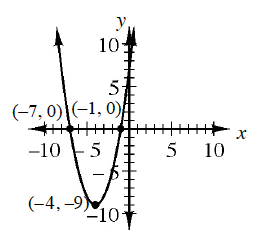### Home > CCAA8 > Chapter 1 Unit 1 > Lesson CCA: 1.2.1 > Problem1-42

1-42.

Use your graph investigation questions from problem 1-32 to describe the graph of the quadratic equation $y=x^2+8x+7$, shown at right.

The graph is a ____________ opening ______ .
The vertex is at (?, ?) and is a minimum/maximum.
There is/is not a line of symmetry. (If so, where?)
The $x$-intercept(s) is/are: ________
The $y$-intercept(s) is/are: ________Use the eTool below to explore.
Click the link at right for the full version of the eTool: CCA 1-42 HW eTool